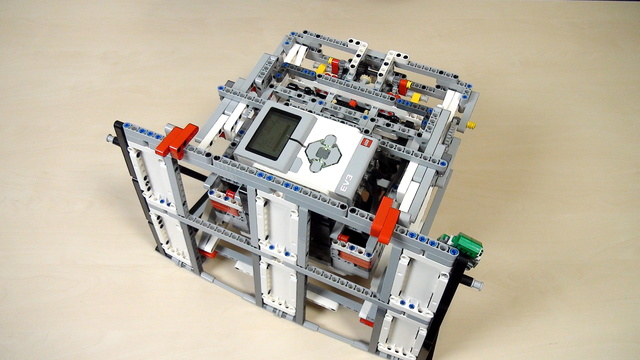# Improving FLL Robot Game. Teacher's Note. Calculating gear rotations ProPreview

What should you do as a teacher when the students are calculating the gear ratios and number of needed rotations?

• #470
• 07 Apr 2017
• 5:02

I would use a sheet of paper with a pencil to discuss the calculations. The math behind this involves just simple division and should be doable by almost all students over 9-10 years old in almost every country of the world.

### Drawing the gear system

You might need to draw the system for the gears for the students. When drawing use the scheme that we are using. It is just a schematic view of the real system. There are a number of ways to represent the system and this on is good enough for the classes that we are doing.

### Multiply the ratios

Draw the system and calculate the ratio between each of the gear wheels. Multiply the ratios and you will get the ratio between the first and the last gear wheel. The number that you should arrive at is 75. This is the ratio of the whole system of this LEGO Mindstorms EV3 gear wheels.

### Students are different age

Try to give the student the task to calculate, but depending on their age you might need to help them a little bit. We leave this up to you, but remember that the students should do more and more of the tasks alone with as little help from you as possible.

### English

This video is for a short teacher notice on calculating the gear ratio from the driving wheel to the driven wheel. The task for the students is to find the number of rotations. Now, how do we approach this? I'll start from the outer wheel. We have here a wheel that has 40 teeth and I'll draw a small scheme of the gear system. And we have a large 40 teeth gear wheel working with a small 8 teeth gear wheel. Then we have this small gear wheel connected to another wheel that has 24 teeth and this is an axle. And here we have a small 8 teeth gear wheel. And looking at the process then it is connected to another 24 teeth gear wheel and it is connected to one more 8 teeth gear wheel. And finally this is connected to a gear wheel with 20 teeth and it is connected with one with 12 teeth. And this is how our system looks. You draw it from the robot to the paper. And then if I just zoom in, what we have are different ratios. We have this gear wheel with 40 teeth gear wheel. I'll add 40 here and we have 8. So, the ratio between the 2 wheels is actually 5. And I'll draw it with 5. And then we have the next. This here is 24 and this here is 8. So, I have here 5 and I have here 3. Then we have another that is 24 and another that is 8 and I have 3 again. And finally, we have the last gear wheel so we have 40/8 = 5, 24/8 = 3, 24/8 = 3 and we have the last one that is 20 by 12. We have 20/12 which is 1.6. So, these are all the ratios between the different gears. 5, 3, 3, 1.6 Now, if we multiply these numbers the number that we arrive to is equal to 75. This means that if we do 75 rotations with this small teeth gear wheel, it will result in one rotation of this large 40 teeth gear wheel. 75 here, 1 here. This is the process in which you calculate. Now, the students might be of different ages. And depending on the group you can enter into these calculations or you can just skip them and try to do more experiments or you can do just a part of these calculations. The important thing is to know the principle, the mechanism, to be able to draw this on the black board or on the white board or on a piece of paper. And it's possible that some of the teams could come up with one ratio, other teams could come up with another ratio. So, it's more like a competition. Who will do the calculation correctly and then to try with this calculation to actually run the robot? So, coming up with different answers from different groups is kind of like a good thing because then you can experiment. You can experiment and see. The answer that we arrived to is 75. But this 75 is for doing 1 full rotation with the driven gear wheel, with this one here. But looking at the construction you cannot do a full rotation. Why? Because if you do a full rotation, you'll break the whole scissor mechanism. What we must do is do 90 degrees. This means 1/4 of the rotation. So, we actually divide this 75 by 4. And this is the answer for rotating the gear wheel the one that is the driving gear wheel to get to a driven wheel that rotates to 90 degrees.

## Courses and lessons with this Tutorial

This Tutorial is used in the following courses and lessons• 48
• 118:45
• 15• 8
• 0
• 2
• 3d_rotation 1